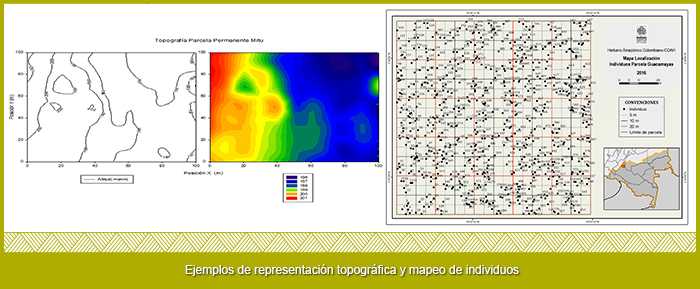# IMPLEMENTATION

Each Permanent Plot of one hectare corresponds to a square of 100x100 meters oriented south north, the selection of the site where the Plot is established follows basic principles of good accessibility and good state of conservation of the forest. Each Permanent Plot established to date has its own characteristics in terms of the owners of the property who determine the exact point where the Parcel is established.

The initial establishment of the plot is done with a precision compass and a decameter with which the limits are demarcated and a correction of the slope is made. The plot is subdivided into 25 quadrants of 20 x 20 meters each. These are delineated with polypropylene rope and in each of the corners with stakes using PVC tubes 1 and 1/2" in diameter and 1m in height, each PVC tube is assigned a plate with the quadrant number ( Figure 2 and 3A).

In turn, each quadrant is subdivided into 16 sub-quadrants of 5 x 5m (Figure 3B).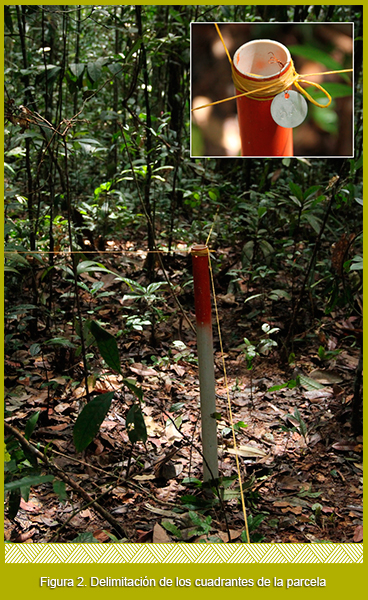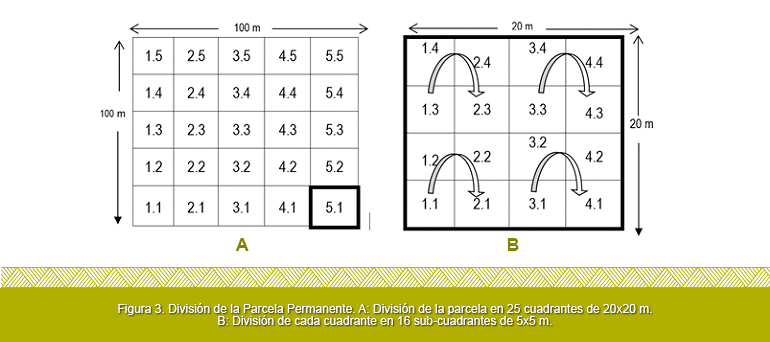Marking of individuals: Each of the trees within the plot is assigned a unique number from a consecutive number of 1 up to the number of trees found. The assigned number is left on the tree with an aluminum sheet plate (Figure 4). The consecutive number assigned to each individual is assigned in clockwise direction of each sub quadrant and, in turn, to the interior of each 10x10 m square (arrows in Figure 3B).

Measurement of specimens: All the trees, arboreal ferns and palms with diameter at breast height (DBH) greater than ten centimeters are measured, the measurement is made at a height of 1.3 m. At the exact site where the DAP measurement is taken, the full circumference is painted with heavy traffic paint (Figure 4). A particular case for the measurement of the DAP of individuals is when they had tabular roots or sneakers, in these cases the measurement is made 50 cm above where the sneakers ended, this requires the use of stairs.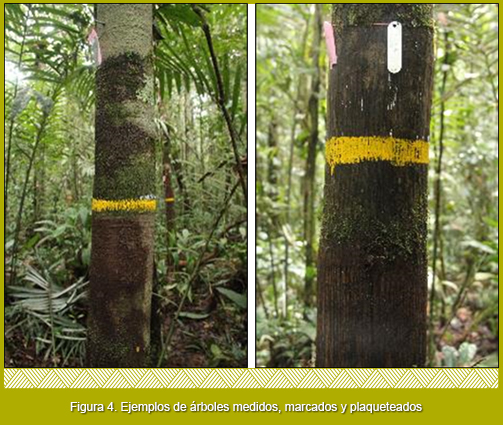Measurement of heights: They are performed with a hypsometer laser for 40% of individuals. And for the total of the individuals it is estimated.

Botanical Collection: To attain the botanical samples, the trees are climbed and a branch is cut through a short branch that is identified with the corresponding number; in the field notebook data that is lost in the botanical collection such as exudates, odors , characteristics of the shaft and/or roots is noted. This exercise is carried out with the help of local experts from the different communities that accompany the establishment of the parcels. The samples obtained are processed according to the standards of the Colombian Amazonian Herbarium COAH of the SINCHI Institute.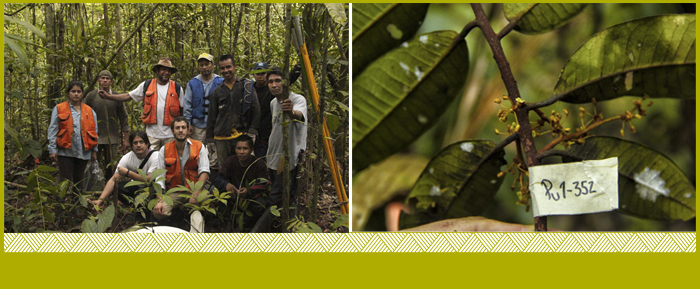Topographic measurement and mapping: In each quadrant the differences in ground heights are measured every ten meters in order to generate a slope map of the plot. In turn, each individual is mapped with the help of sub-divisions made within each quadrant.